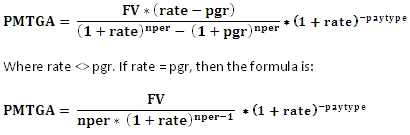# SQL Server PMT function for a growing annuity

PMTGA

Updated: 07 February 2011

Use PMTGA to calculate the initial payment for a growing annuity, given the future value. The formula for the calculation of the initial payment of a growing annuity is:Syntax
SELECT [wctFinancial].[wct].[PMTGA](
<@FV, float,>
,<@Pgr, float,>
,<@Nper, int,>
,<@Rate, float,>
,<@Pay_type, int,>)
Arguments
@FV
the future value of the annuity. @FV is an expression of type float or of a type that can be implicitly converted to float.
@Pgr
the periodic growth rate of the annuity. This is the percentage amount, expressed as a decimal, by which the annuity will increase in each period. @Pgr is an expression of type float or of a type that can be implicitly converted to float.
@Nper
the number of annuity payments. @Nper is an expression of type float or of a type that can be implicitly converted to float.
@Rate
the percentage rate of return, expressed as a decimal, that you expect the annuity to earn over the number of periods. The annuity payments are compounded using this value. @Rate is an expression of type float or of a type that can be implicitly converted to float.
@Pay_type
the number 0 or 1 and indicates when payments are due. @Pay_type is an expression of type int or of a type that can be implicitly converted to int. If @Pay_type is not 0 it is assumed to be 1.

 Set @Pay_type equal to If payments are due 0 At the end of a period 1 At the beginning of a period

Return Type
float
Remarks
·         The PMTGA value will have the same sign as @FV.
·         If the @Pay_type is not equal to zero, it is assumed to be 1.
·         To calculate the Future value of a growing annuity, use the FVGA function.
Examples
Let’s say you are going to work for 40 more years, and you would like to have a million dollars in your tax-deferred account after 40 years. Assuming that you can increase your contributions by 3% per year and that your tax-deferred account will earn 7% per year, what is the initial (annual) payment to be made into the annuity?
SELECT
wct.PMTGA(1000000 --@FV
,.03        --@Pgr
,40         --@Nper
,.07        --@Rate
,1          --@Pay_type
) as PMT
This produces the following result.
PMT
----------------------
3191.75519827819

(1 row(s) affected)

We can verify this calculation by using the FVGA function.
SELECT wct.FVGA(3191.75519827819,.03,40,.07,1) as FVGA

This produces the following result.
FVGA
----------------------
1000000

(1 row(s) affected)

### SupportCopyright 2008-2021 Westclintech LLC         Privacy Policy        Terms of Service